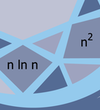# Parameterized and Exact Algorithms#### This series of talks was part of the Fine-Grained Complexity and Algorithm Design Boot Camp. Videos for each talk area available through the links above.

We expect that NP-hard problems can be solved only by algorithms that need exponential time in the worst case. However, there is still a lot that can be said about the complexity of such problems. The study of exponential algorithms aims to find the best possible form of exponential running time that can be achieved. Parameterized complexity analyzes the running time of an algorithm as a function of the input size n and some parameter k of the input. The goal is to determine the optimal dependence on the parameter. Of particular interest is the case when only a multiplicative factor of the running time depends on the parameter k, that is, the problem is fixed-parameter tractable (FPT).

The mini-course will survey a selection of algorithmic results, highlighting some nontrivial algorithmic techniques for NP-hard problems. Then we show how the (Strong) Exponential-Time Hypothesis and other conjectures can be used to give lower bounds that, in many cases, tightly match the algorithmic results.